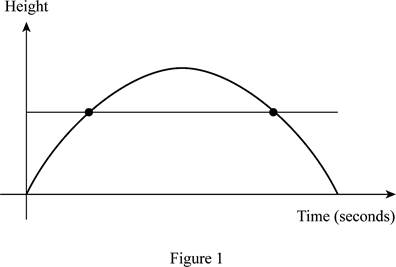# Whether the function which reflects the height of the football at t seconds after kickoff.### Single Variable Calculus: Concepts...

4th Edition
James Stewart
Publisher: Cengage Learning
ISBN: 9781337687805### Single Variable Calculus: Concepts...

4th Edition
James Stewart
Publisher: Cengage Learning
ISBN: 9781337687805

#### Solutions

Chapter 1.6, Problem 13E
To determine

## Whether the function which reflects the height of the football at t seconds after kickoff.

Expert Solution

The function is not a one-to one function.

### Explanation of Solution

The given function is, f(t)=Height of the football t s after kickoff .

It is known that the football will reach ground in few seconds after kickoff. And hence the foot ball will reach the same height at two different times. Thus, the graph of the function f(t)=Height of the football t s after kickoff is shown below in Figure 1.From Figure 1, it is observed that the horizontal line intersects the curve at two points, which means it fails the horizontal line test. Thus, it can be concluded that the function f(t)=Height of the football t s after kickoff is not a one-to-one function.

### Have a homework question?

Subscribe to bartleby learn! Ask subject matter experts 30 homework questions each month. Plus, you’ll have access to millions of step-by-step textbook answers!